# Prediction using ColumnTransformer, OneHotEncoder and Pipeline

In this tutorial, we’ll predict insurance premium costs for each customer having various features, using ColumnTransformer, OneHotEncoder and Pipeline.
We’ll import the necessary data manipulating libraries:
Code:

 `import` `pandas as pd ` `import` `numpy as np ` ` `  `from` `sklearn.compose ``import` `ColumnTransformer ` `from` `sklearn.model_selection ``import` `train_test_split, cross_val_score ` `from` `sklearn.impute ``import` `SimpleImputer ` `from` `sklearn.preprocessing ``import` `OneHotEncoder, StandardScaler, MinMaxScaler ` `from` `sklearn.pipeline ``import` `Pipeline ` `from` `sklearn.ensemble ``import` `RandomForestRegressor `

We’ll now load the dataset, which is available here:
Each row is a different individual, having an age, gender, body mass index (bmi), number of dependents, whether they smoke, the region from where they belong, and the insurance premium they pay.

Code:

 `df ``=` `pd.read_csv(``'https://raw.githubusercontent.com / stedy / Machine-Learning-with-R-datasets / master / insurance.csv'``) ` `df.head() `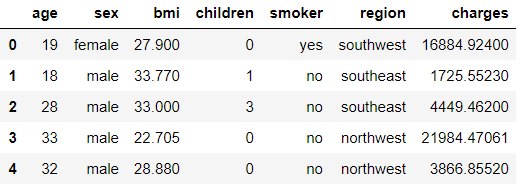Code:

 `df.info() `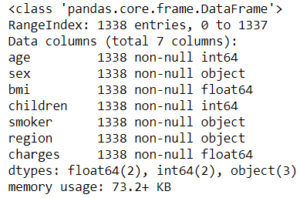Code:

 `df.isna().``sum``() `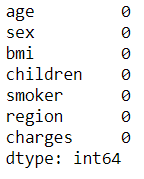We see there are none. But we will introduce ‘impurities’ in this dataset just because a smooth sea has never made a skilled sailor! … apart from the fact that we need missing values to demonstrate ColumnTransformer in a better way.

Code:

 `np.random.seed(``0``) ``# for reproducibility ` `for` `_ ``in` `range``(``10``): ` `    ``r ``=` `np.random.randint(``len``(df)) ` `    ``c ``=` `np.random.randint(``6``) ` `    ``df.iloc[r, c] ``=` `np.nan `

With range(10) we imply that we need NaN’s at 10 places in the data, whether each NaN in a different row or multiple NaNs in a row, we won’t mind.

Code:

 `df.isna().``sum``() `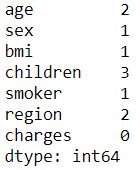We’ll now split the data into train and test sets.

Code:

 `X_train, X_test, y_train, y_test ``=` `train_test_split(df.drop(``'charges'``, ``1``), ` `                                                    ``df[``'charges'``], ` `                                                    ``test_size ``=` `0.2``, random_state ``=` `0``) `

Now enters the ColumnTransformer!
A ColumnTransformer takes in a list, which contains tuples of the transformations we wish to perform on the different columns. Each tuple expects 3 comma-separated values: first, the name of the transformer, which can be practically anything (passed as a string), second is the estimator object, and the final one being the columns upon which we wish to perform that operation.

Code:

 `trf1 ``=` `ColumnTransformer(transformers ``=``[ ` `    ``(``'cat'``, SimpleImputer(strategy ``=``'most_frequent'``), [``'sex'``, ``'smoker'``, ``'region'``]), ` `    ``(``'num'``, SimpleImputer(strategy ``=``'median'``), [``'age'``, ``'bmi'``, ``'children'``]), ` `     `  `], remainder ``=``'passthrough'``) `

First, we’ll impute the categorical columns. We’ll use the most_frequent, or the ‘mode’ type of imputation, and the categorical columns are ‘sex’, ‘smoker’ and ‘region’. We’ll name this transformer ‘cat’ for simplicity.
Similarly we’ll do the imputation of the numerical columns using medians of respective columns. We now need to tell the ColumnTransformer what it should do with the remaining columns, i.e. the columns upon which no transformation was performed. In our case, all features are used, but in cases were you have ‘unused’ columns, you can specify whether you want to drop or retain those columns after the transformation. We’ll retain them, hence pass remainder=’passthrough’ instead of the default behavior which is to drop those columns. We could have also specified the columns as their integer positions instead of their names, like for [‘age’, ‘bmi’, ‘children’], we could’ve said [0, 2, 3] etc. Now we’ll fit and transform the X_train to see the output, which is a numpy array by default:
Code:

 `first_step ``=` `trf1.fit_transform(X_train) ` `first_step `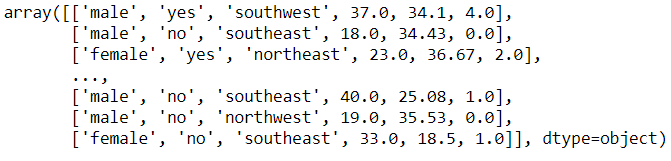We’ll make a data frame out of it:
Code:

 `pd.DataFrame(first_step).head() `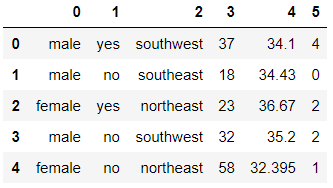Did you notice that the columns have been reordered, and the column names are now lost? They’ve been reordered in the order of the transformers that we passed to the ColumnTransformer, i.e. we first asked it to impute the categorical columns, hence they’ve been placed first, and so on…

Code:

 `pd.DataFrame(first_step).isna().``sum``() `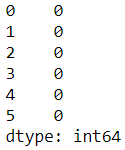We can check what each transformer is doing by using the ‘names’ we passed in the tuples:
Code:

 `trf1.named_transformers_ ` `# this is a dictionary, with the names of the transformers as keys. `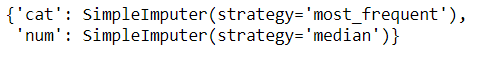Code:

 `trf1.named_transformers_[``'num'``].statistics_ ` `# you see, these were the median values of each of the three numerical columns. ` `# for any transformer, you can access its specific attributes this way. `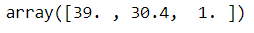Now that all columns are free of missing values, we can go ahead with encoding of the categorical columns.

Note: OneHotEncoder can’t handle missing values, hence it is important to get rid of them before encoding. Now, we make another transformer object for the encoding. We couldn’t do this in ‘trf1’ because at that point in time, there were missing values in the X_train, and OneHotEncoder can’t deal with missing values as discussed earlier. Hence we first needed to remove the missing values, and then pass this new ‘first_step’ array (with no missing values) to OneHotEncoder.

Code:

 `trf2 ``=` `ColumnTransformer(transformers ``=``[ ` `    ``(``'enc'``, OneHotEncoder(sparse ``=` `False``, drop ``=``'first'``), ``list``(``range``(``3``))), ` `], remainder ``=``'passthrough'``) `

We set the sparse parameter to False (because we want a dense array output) and we can toggle between dropping the first of the dummy encoded columns or not, depending upon the type of model we’re fitting, to avoid the ‘dummy variable trap’. Learn more about it here: A general rule of thumb: drop a dummy-encoded column if using a linear-based model, and do not drop it if using a tree-based model. Also, did you see how for the columns parameter, we specified list(range(3)) instead of the column names? That is because now, we’ve lost the column names (as seen in ‘first_step’, but we know the categorical columns are the first three columns (after reordering), hence we specify [0, 1, 2].

Code:

 `second_step ``=` `trf2.fit_transform(first_step) ` `pd.DataFrame(second_step).head() ` ` `  `# Now we have our one hot encoded data ! Sweet ! `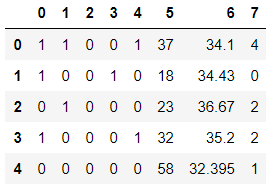Now comes the Pipeline! We could’ve performed all these steps in one single Pipeline instance. The pipeline also expects a list of tuples, and each tuple in turn expecting two values: name of the step and the object.

Code:

 `pipe ``=` `Pipeline(steps ``=``[ ` `    ``(``'tf1'``, trf1), ` `    ``(``'tf2'``, trf2), ` `    ``(``'tf3'``, MinMaxScaler()), ``# or StandardScaler, or any other scaler ` `    ``(``'model'``, RandomForestRegressor(n_estimators ``=` `200``)), ` `# or LinearRegression, SVR, DecisionTreeRegressor, etc ` `]) `

Code:

 `# we'll use cross_val_score with 5 splits to better examine our model. ` `# we'll send our entire 'pipe' object to the cross_val_score and it will take ` `# care of all the preprocessing work for us ! cvs = cross_val_score(pipe, X_train, y_train, cv = 5) ` `print``(``"All cross val scores:"``, cvs) ` `print``(``"Mean of all scores: "``, cvs.mean()) `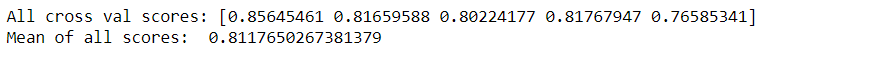So our model is around 81.2% accurate. You could try different regressors, tweak parameters, use StandardScaler or other scalers, and see if you can achieve better results. We can use GridSearchCV to do this work of finding best set of parameters for us. We’ll now fit the model on the entire training set, and predict results on the test set:

Code:

 `pipe.fit(X_train, y_train) `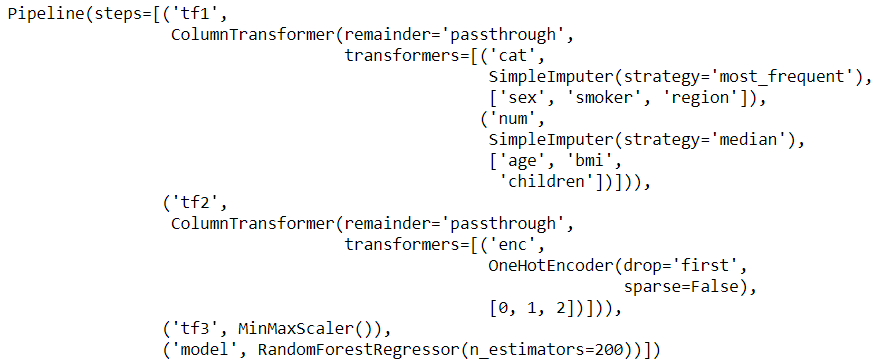Code:

 `preds ``=` `pipe.predict(X_test) ` ` `  `# This is how the original test set insurance prices and  ` `# our predicted ones stack up ` ` `  `pd.DataFrame({``'original test set'``:y_test, ``'predictions'``: preds}) `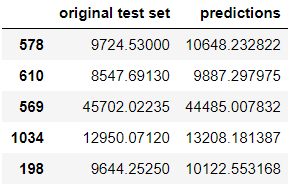My Personal Notes arrow_drop_upCheck out this Author's contributed articles.

If you like GeeksforGeeks and would like to contribute, you can also write an article using contribute.geeksforgeeks.org or mail your article to contribute@geeksforgeeks.org. See your article appearing on the GeeksforGeeks main page and help other Geeks.

Please Improve this article if you find anything incorrect by clicking on the "Improve Article" button below.

Article Tags :
Practice Tags :

Be the First to upvote.

Please write to us at contribute@geeksforgeeks.org to report any issue with the above content.